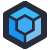Last week, I was doing some graphics programming without a graphics card. It was low resolution, so I went ahead and re-implemented a few key methods from the Open GL Shader Language in a fashion which was compatible with NumPy arrays. Lucky for me, I was able to draw off many years of experience, I understood both technologies, and they both have excellent documentation which made it easy. After dozens of lines of code, I was able to whip up some pretty flexible image generator functions. I knew the tools I needed, I understood how they worked, and while I was reinventing a wheel, I had a very specific reason.

Philemon Eichin sends us some code from a point in his career where none of these things were true.

Philemon was building a changelog editor. As such, he wanted an easy, flexible way to identify patterns in the text. Philemon knew that there was something that could do that job, but he didn’t know what it was called or how it was supposed to work. So, like all good programmers, Philemon went ahead and coded up what he needed- he invented his own regular expression language, and built his own parser for it.

Thus was born Philegex. Philemon knew that regexes involved slashes, so in his language you needed to put a slash in front of every character you wanted to match exactly. He knew that it involved question marks, so he used the question mark as a wildcard which could match any character. That left the ’|" character to be optional.

So, for example: `/P/H/I/L/E/G/E/X|???` would match “PHILEGEX!!!” or “PHILEGEWTF”. A date could be described as: `nnnn/.nn/.nn`. (YYYY.MM.DD or YYYY.DD.MM)

Living on his own isolated island without access to the Internet to attempt to google up “How to match patterns in text”, Philemon invented his own language for describing parts of a regular expression. This will be useful to interpret the code below.

PhilegexRegex
p1 Pattern / Regex
Block(s)Token(s)
CT CharType
SplitLineParseRegex
CC currentChar
auf_zu openParenthesis
Chars CharClassification

With the preamble out of the way, enjoy Philemon’s approach to regular expressions, implemented elegantly in VB.Net.

``````Public Class Textmarker
Const Datum As String = "nn/.nn/.nnnn"

Private Structure Blocks
Dim Type As Chars
Dim Multi As Boolean
Dim Mode As Char_Mode
Dim Subblocks() As Blocks
Dim passed As Boolean
Dim _Optional As Boolean
End Structure

Public Shared Function IsMaskable(p1 As String, Content As String) As Boolean
Dim ID As Integer = 0
Dim p2 As Chars
Dim _Blocks() As Blocks = SplitLine(p1)
For i As Integer = 0 To Content.Length - 1
p2 = GetCT(Content(i))
START_CASE:
'#If CONFIG = "Debug" Then
'            If ID = 2 Then
'                Stop
'            End If

'#End If
If ID > _Blocks.Length - 1 Then
Return False
End If
Select Case _Blocks(ID).Mode
Case Char_Mode._Char
If p2.Char_V = _Blocks(ID).Type.Char_V Then
_Blocks(ID).passed = True
If Not _Blocks(ID).Multi = True Then ID += 1
Exit Select
Else
If _Blocks(ID).passed = True And _Blocks(ID).Multi = True Then
ID += 1
GoTo START_CASE
Else
If Not _Blocks(ID)._Optional Then Return False

End If
End If
Case Char_Mode.Type
If _Blocks(ID).Type.Type = Chartypes.any Then
_Blocks(ID).passed = True
If Not _Blocks(ID).Multi = True Then ID += 1
Exit Select
Else

If p2.Type = _Blocks(ID).Type.Type Then
_Blocks(ID).passed = True
If Not _Blocks(ID).Multi = True Then ID += 1
Exit Select
Else
If _Blocks(ID).passed = True And _Blocks(ID).Multi = True Then
ID += 1
GoTo START_CASE
Else
If _Blocks(ID)._Optional Then
ID += 1
_Blocks(ID - 1).passed = True
Else
Return False

End If

End If
End If

End If

End Select

Next
For i = ID To _Blocks.Length - 1
If _Blocks(ID)._Optional = True Then
_Blocks(ID).passed = True
Else
Exit For
End If
Next
If _Blocks(_Blocks.Length - 1).passed Then
Return True
Else
Return False
End If

End Function

Private Shared Function GetCT(Char_ As String) As Chars

If "0123456789".Contains(Char_) Then Return New Chars(Char_, 2)
If "qwertzuiopüasdfghjklöäyxcvbnmß".Contains((Char.ToLower(Char_))) Then Return New Chars(Char_, 1)
Return New Chars(Char_, 4)
End Function

Private Shared Function SplitLine(ByVal Line As String) As Blocks()
Dim ret(0) As Blocks
Dim retID As Integer = -1
Dim CC As Char
For i = 0 To Line.Length - 1
CC = Line(i)
Select Case CC
Case "("
ReDim Preserve ret(retID + 1)
retID += 1
Dim ii As Integer = i + 1
Dim auf_zu As Integer = 1
Do
Select Case Line(ii)
Case "("
auf_zu += 1
Case ")"
auf_zu -= 1
Case "/"
ii += 1
End Select
ii += 1
Loop Until auf_zu = 0
ret(retID).Subblocks = SplitLine(Line.Substring(i + 1, ii - 1))
ret(retID).Mode = Char_Mode.subitems
ret(retID).passed = False

Case "*"
ret(retID).Multi = True
ret(retID).passed = False
Case "|"
ret(retID)._Optional = True

Case "/"
ReDim Preserve ret(retID + 1)
retID += 1
ret(retID).Mode = Char_Mode._Char
ret(retID).Type = New Chars(Line(i + 1), Chartypes.other)
i += 1
ret(retID).passed = False

Case Else

ReDim Preserve ret(retID + 1)
retID += 1
ret(retID).Mode = Char_Mode.Type
ret(retID).Type = New Chars(Line(i), TocType(CC))
ret(retID).passed = False
End Select
Next
Return ret
End Function
Private Shared Function TocType(p1 As Char) As Chartypes
Select Case p1
Case "c"
Return Chartypes._Char
Case "n"
Return Chartypes.Number
Case "?"
Return Chartypes.any
Case Else
Return Chartypes.other
End Select
End Function

Public Enum Char_Mode As Integer
Type = 1
_Char = 2
subitems = 3
End Enum
Public Enum Chartypes As Integer
_Char = 1
Number = 2
other = 4
any
End Enum
Structure Chars
Dim Char_V As Char
Dim Type As Chartypes
Sub New(Char_ As Char, typ As Chartypes)
Char_V = Char_
Type = typ
End Sub
End Structure
End Class``````

I’ll say this: building a finite state machine, which is what the core of a regex engine is, is perhaps the only case where using a `GoTo` could be considered acceptable. So this code has that going for it. Philemon was kind enough to share this code with us, so we knew he knows it’s bad.[Advertisement] ProGet can centralize your organization's software applications and components to provide uniform access to developers and servers. Check it out!